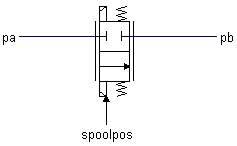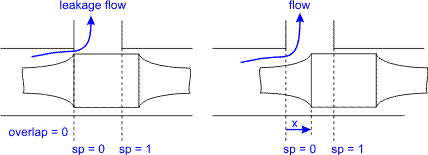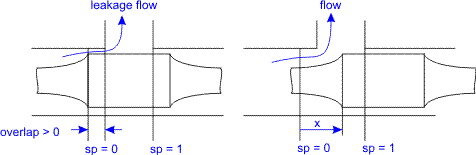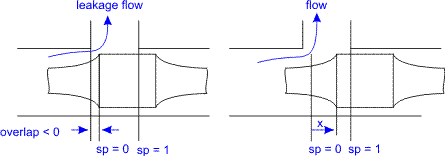﻿ 20-sim webhelp > Library > Iconic Diagrams > Hydraulics > Valves > Basic Valves > twotwowayproportionalvalve

# twotwowayproportionalvalve

Navigation:  Library > Iconic Diagrams > Hydraulics > Valves > Basic Valves >

# twotwowayproportionalvalve## Library

Iconic Diagrams\Hydraulics\Valves\Basic Valves

## Use

Domains: Continuous. Size: 1-D. Kind: Iconic Diagrams (Hydraulics).

## DescriptionThis model describes a 2/2-way proportional control valve with second order spool dynamics. The flow through the valve is described as laminar/turbulent flow through an orifice:

dp = pa.p - pb.p

phi = sign(dp) * Cd * A(sp) * sqrt( (2/rho) * abs(dp) ) + GLeak * dp;

Here Cd is the discharge coefficient which normally has a value between 0.55 and 0.7. A(sp) is the area of the orifice opening. A(sp) depends linearly on the spool position sp and varies between 0 (sp = 0) and the maximum area Amax (sp = 1). Gleak is the conductance of laminar leakage flow when the valve is closed. The relative opening of the spool valve is indicated by sp. For a closed valve the spool position (sp) is equal to zero and for an open valve the spool position (sp) is equal to 1.In the neutral spool position (sp = 0) the valve is just closed. A positive overlap indicates that the spool must travel a certain distance before the valve opens.A negative overlap indicates that the valve is already open in the neutral position.The overlap is indicated by the parameter overlap, which is given as a fraction of the spool position.

The spool position sp is a function of the input signal spoolpos:

sp = SO(f,d,spoolpos)

where SO is a second order transfer function to model the spool dynamics. The function is characterized by the bandwidth (f) and damping (d). The valve input position spoolpos should be limited to the range between 0 and 1.

The pressure at both ports has a lower limit which is equal to the vapour pressure. Therefore the actual equations used in this component are:

pa_lim = if pa.p < p_vapour then p_vapour else pa.p end;

pb_lim = if pb.p < p_vapour then p_vapour else pb.p end;

## Interface

 Ports Description pa, pb Both terminals of the valve. Causality fixed volume flow out pa fixed volume flow out pb Inputs spoolpos position of the spool valve: 0 =closed, 1 = open 0 <= spoolpos <= 1
 Parameters Q_nom Nominal flow at nominal pressure per edge, spool in the open position [m3/s], Q_nom > 0 Q_leak p_nom overlap f Leakage flow at nominal pressure per edge [m3/s], Q_leak > 0 Nominal pressure per edge [Pa], p_nom > 0 Valve overlap as  percentage of full stroke, -1 < overlap < 1. Natural frequency, f > 0.